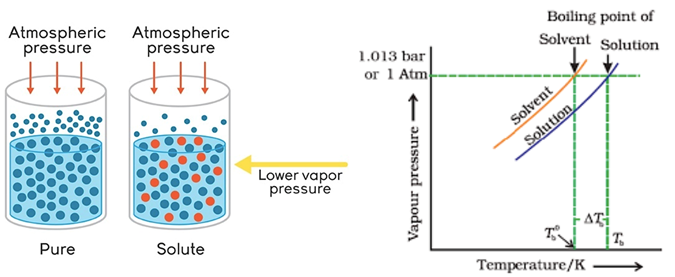# Boiling Point Formula

## Boiling Point Formula - Boiling Point Elevation Formula & Solved Examples

By definition, the boiling point is the temperature at which the vapor pressure of the liquid equals the surrounding pressure and liquid turns into vapor. The phenomenon of boiling is pressure dependent and hence the boiling point of a liquid may change depending upon the surrounding pressure. For example, due to the change in atmospheric pressure at different altitude, water boils at 100 °C (212 °F) at sea level, but at 93.4 °C (200.1 °F) at 1,905 meters (6,250 ft). For a given pressure, different liquids will boil at different temperatures.

Elevation in boiling point:
We know that pure water boils at 100 °C at 1 atm pressure but an interesting thing happens to the boiling point if we add a small amount of salt to water. It has been experimentally proven that adding any form of non-volatile solute to a liquid increases its boiling point. The amount by which the boiling point changes is directly proportional to the amount of solute added.Let T0b denote boiling point of pure liquid, and Tb denote the boiling point of solution (solute + pure liquid). Then,
$\Delta {T_b}\,\alpha \,m$
$\Delta {T_b} = {K_b} \times m$
Where, $\Delta {T_b} = T_b^0 - {T_b}$ is elevation in boiling point,
m is the molality,
‘${K_b}$’ is the molal elevation, boiling point or ebullioscopic constant whose value depends only on the solvent
The equation can further be written as,

$\Delta {T_b} = \frac{{1000 \times {K_b} \times w}}{{M \times W}}$ where, ‘’ is weight of the solute, ‘’ is the molar mass of the solute and ‘W’ is the weight of the solvent in grams.

Example: What is the boiling point elevation when 147 g of lactic acid (C6H10O5) is dissolved in 647 g of cyclohexane (C6H12)? The boiling point constant for cyclohexane is 2.79 °C/m.
Solution:
Determine the molality of the lactic acid solution
m = (147 g / 162.14 g/mol) / 0.647 kg = 1.40127
$\Delta {T_b} = {K_b} \times m$
$\Delta {T_b} =$ (2.79 °C kg mol-1) (1.40127 mol/kg)
$\Delta {T_b}$ = 3.91 °C

Question: A solution of 10.0 g of a nonvolatile, non-dissociating compound dissolved in 0.200 kg of benzene boils at 81.2 °C. Calculate the molecular weight of the compound. Ebullioscopic constant for benzene: 2.53 °C/m and boiling point of pure benzene: 80.1°C
Options:
(a) 115 gm/mol
(b) 145 gm/mol
(c) 120 gm/mol
(d) 100 gm/mol
$\Delta {T_b} = \frac{{1000 \times {K_b} \times w}}{{M \times W}}$
$1.1 = \frac{{1000 \times 2.53 \times 10}}{{M \times 200}}$